Ask your WordPress questions! Pay money and get answers fast!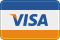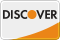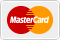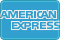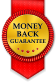# Function to Filter genesis_get_image with get_the_image Plugin WordPress

• SOLVED

Hi all,

I'm close to a solution, but need help to finish up. I am using the "News" Child Theme on the Genesis framework by StudioPress. Basically I have to filter the Genesis call to the images with the Get the Image plugin by Justin Tadlock. Read below to see why...

Here's some relevant information from my StudioPress forum posts.

My original post:
<blockquote>I'm looking to accomplish the same thing...grab the first image from an RSS feed being brought in by FeedWordPress and add that image as a post thumbnail.

In case you're wondering, here's my use case. I have a client with a small network of pet related sites. They are adding unique hand written content to all of the sites, but they have a "General News" category on the main install that they want to "feed" to the other sites.

I am using the News Child Theme and everything works great except no post thumbnails</blockquote>

Then after a little more research and learning I needed to filter the Genesis image call with the Get the Image plugin, I found a function:
http://www.studiopress.com/support/showpost.php?p=198098&postcount=10
``` add_filter('genesis_get_image', 'default_image_fallback', 10, 2); function default_image_fallback(\$output, \$args) { if( !\$output && \$args['size'] == 'thumbnail' && \$args['format'] == 'html' && function_exists( 'get_the_image' ) ) \$output = get_the_image(array( 'size' => 'thumbnail', 'image_scan' => 'true', 'width' => '150', 'height' => '150', 'image_class' => 'post-image' )); return \$output; } ```
<blockquote>I just realized that the Featured Tabs and Featured Posts had different image sizes chosen. I changed the Featured Posts to call the Thumbnail (150x150) size and all the thumbs showed up. However...a couple things...

1. The thumbnails that do show are above the content, even though I have align left chosen in the Featured Posts widget.

2. In order for this function to work with all aspects of the News Child Theme, the other sizes need to be added. Or would I need to add a separate function for each size?</blockquote>

In other words, the above function works for the thumbnail size, but not the other sizes that Genesis allows in this theme. I tried adding another size by using the following function, but it doesn't work.
``` add_filter('genesis_get_image', 'default_image_fallback', 10, 2); function default_image_fallback(\$output, \$args) { if( !\$output && \$args['size'] == 'thumbnail' && \$args['format'] == 'html' && function_exists( 'get_the_image' ) ) \$output = get_the_image(array( 'size' => 'thumbnail', 'image_scan' => 'true', 'width' => '150', 'height' => '150', 'image_class' => 'post-image' )); else if( !\$output && \$args['size'] == 'square' && \$args['format'] == 'html' && function_exists( 'get_the_image' ) ) \$output = get_the_image(array( 'size' => 'square', 'image_scan' => 'true', 'width' => '110', 'height' => '110', 'image_class' => 'post-image' )); return \$output; } ```

1. I'm looking for a function that will work for all sizes that Genesis provides in this theme.
2. Related would be an answer to why the alignment of the image is above the content instead of to the left as it should be.

An example can be seen here: http://mybostondog.oneworldpet.com/category/test/

2010-10-02

I don't really have enought time right now to take a closer look at this, but your conditional statement syntax is kinda wrong. Try using this one:
```add_filter('genesis_get_image', 'default_image_fallback', 10, 2); function default_image_fallback(\$output, \$args) { if(!\$output && \$args['size'] == 'thumbnail' && \$args['format'] == 'html' && function_exists( 'get_the_image' )){ \$output = get_the_image(array( 'size' => 'thumbnail', 'image_scan' => 'true', 'width' => '150', 'height' => '150', 'image_class' => 'post-image' )); } elseif (!\$output && \$args['size'] == 'square' && \$args['format'] == 'html' && function_exists( 'get_the_image' )){ \$output = get_the_image(array( 'size' => 'square', 'image_scan' => 'true', 'width' => '110', 'height' => '110', 'image_class' => 'post-image' )); } else { \$output = 'default output'; } return \$output; }```

I think this got me closer, and yes, I'm certain my syntax was off;) I tried this and the first function worked as expected for the thumbnail, but the square image didn't get pulled in or filtered. Where the square image should be, there is a link to "default output" that links to the full post.

Do you have any thoughts on what may be happening?

```add_filter('genesis_get_image', 'default_image_fallback', 10, 2); function default_image_fallback(\$output, \$args) { if(!\$output && \$args['size'] == 'thumbnail' && \$args['format'] == 'html' && function_exists( 'get_the_image' )){ \$output = get_the_image(array( 'size' => 'thumbnail', 'image_scan' => 'true', 'width' => '150', 'height' => '150', 'image_class' => 'post-image' )); } else { \$output = get_the_image(array( 'size' => 'square', 'image_scan' => 'true', 'width' => '110', 'height' => '110', 'image_class' => 'post-image' )); } return \$output; }```

what about something like this? ;)

Thank you, that works great! However, I do need to add two more image sizes. I tried to add one and my lack of PHP syntax knowledge is showing again...after I added the "Mini Square" size I am getting an Parse error: syntax error, unexpected T_ELSE error on the the line that holds the ` } else {` above the Mini Square code.

Either way, you win, but could you help me out with that syntax again?

``` add_filter('genesis_get_image', 'default_image_fallback', 10, 2); function default_image_fallback(\$output, \$args) { if(!\$output && \$args['size'] == 'thumbnail' && \$args['format'] == 'html' && function_exists( 'get_the_image' )){ \$output = get_the_image(array( 'size' => 'thumbnail', 'image_scan' => 'true', 'width' => '150', 'height' => '150', 'image_class' => 'post-image' )); } else { \$output = get_the_image(array( 'size' => 'square', 'image_scan' => 'true', 'width' => '110', 'height' => '110', 'image_class' => 'post-image' )); } else { \$output = get_the_image(array( 'size' => 'Mini Square', 'image_scan' => 'true', 'width' => '70', 'height' => '70', 'image_class' => 'post-image' )); } return \$output; } ```

``` add_filter('genesis_get_image', 'default_image_fallback', 10, 2); function default_image_fallback(\$output, \$args) { if(!\$output && \$args['size'] == 'thumbnail' && \$args['format'] == 'html' && function_exists( 'get_the_image' )){ \$output = get_the_image(array( 'size' => 'thumbnail', 'image_scan' => 'true', 'width' => '150', 'height' => '150', 'image_class' => 'post-image' )); } elseif (!\$output && \$args['size'] == 'Mini Square' && \$args['format'] == 'html' && function_exists( 'get_the_image' )) { \$output = get_the_image(array( 'size' => 'Mini Square', 'image_scan' => 'true', 'width' => '70', 'height' => '70', 'image_class' => 'post-image' )); } else { \$output = get_the_image(array( 'size' => 'square', 'image_scan' => 'true', 'width' => '110', 'height' => '110', 'image_class' => 'post-image' )); } return \$output; }```

if/elseif/else statements works like this:
``` if(condition1) { condition passed so this will be done } elseif (condition2) { condition1 dont pass, so we check for condition2. if it passes - this will be done. } else { nothing passed so we echo this out. }```

So, in your situation, we check if \$args['size'] is equal to 'thumbnail'. If it IS equal - output will be 'thumbnail' image. If that argument wasn't equal to 'thumbnail', then we check if it is equal to 'Mini Square'. If it is - we form output with 'Mini Square' sized image. Else, if that argument was not equal to 'thumbnail', nor 'Mini Square' we default it to 'square' image ;) I hope that helps ;)

btw, this should also work, and might be easier for you to understand.

``` add_filter('genesis_get_image', 'default_image_fallback', 10, 2); function default_image_fallback(\$output, \$args) { if(!\$output && \$args['size'] == 'thumbnail' && \$args['format'] == 'html' && function_exists( 'get_the_image' )){ \$output = get_the_image(array( 'size' => 'thumbnail', 'image_scan' => 'true', 'width' => '150', 'height' => '150', 'image_class' => 'post-image' )); } if(!\$output && \$args['size'] == 'Mini Square' && \$args['format'] == 'html' && function_exists( 'get_the_image' )) { \$output = get_the_image(array( 'size' => 'Mini Square', 'image_scan' => 'true', 'width' => '70', 'height' => '70', 'image_class' => 'post-image' )); } if(!\$output && \$args['size'] == 'square' && \$args['format'] == 'html' && function_exists( 'get_the_image' )){ \$output = get_the_image(array( 'size' => 'square', 'image_scan' => 'true', 'width' => '110', 'height' => '110', 'image_class' => 'post-image' )); } return \$output; }```

Simple if statements to check which condition will pass. Depends on \$args['size'] ;)
So now, when you want to add any other image size, just add something like this to the function above:

``` if(!\$output && \$args['size'] == 'somenewsize' && \$args['format'] == 'html' && function_exists( 'get_the_image' )){ \$output = get_the_image(array( 'size' => 'somenewsize', 'image_scan' => 'true', 'width' => '110', 'height' => '110', 'image_class' => 'post-image' )); } ```

As an aside, the last one (the easier one to understand) worked for thumbnail and Mini Square, but not square. The one above worked for all three image sizes.

2010-10-02

can you tell me how do you call the get image of genesis?

regarding the alignment as i see in your site there is no alignleft class on the image, it should be i guess like this:

``` \$output = get_the_image(array( 'size' => 'thumbnail', 'image_scan' => 'true', 'width' => '150', 'height' => '150', 'image_class' => 'post-image alignleft' )); ```

2010-10-03